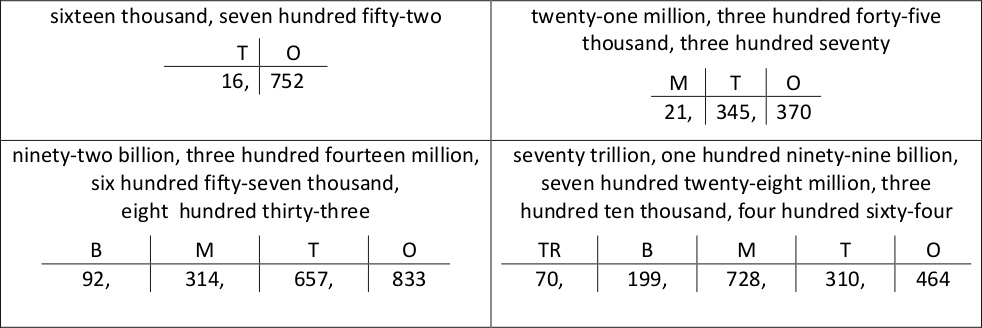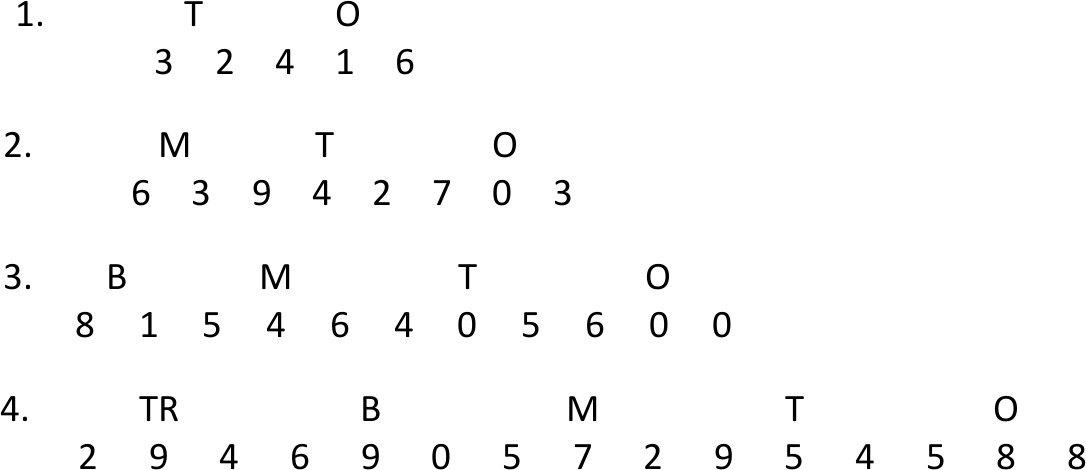### How to Say and Write Large Numbers

Reading large numbers can be difficult for some students. Fortunately, numbers are grouped in three digits called periods that are separated by commas to make saying and reading large numbers more manageable. Periods make the process of saying numbers easy. The order of the periods from least to greatest is as follows: ones, thousand, million, billion, trillion. Make the following points:

• These periods are not followed by an “s.”
• A comma separates every three-digit period.
• The largest period of a number can have 1-, 2-, or 3- digits.
• The other periods that follow the largest period must have three digits.
• It should be very easy to say large numbers if students can say one, two, and three-digit numbers such as 3, 56, and 642.

Display several one-, two-, and three-digit numbers like the ones below. Call on individual students to recite the numbers.

12      23      455      9      708      950

Review the names of the periods in order from right to left, which is from the smallest period to the largest period. Memorizing the least to greatest order and the greatest to least order can benefit students

trillion    |    billion    |    million    |    thousand    |    ones

Write the underlined letters from each period using the following abbreviations: O, T, M, B,TR.

Call attention to the two periods that begin with the letter “T”. Explain that the trillion’s period will be identified with the letters “TR” for students to tell the difference between the thousand and trillion periods. Have students recall the abbreviations for the periods in least to greatest order (O, T, M, B, or TR).

Remind students to make sure that they label periods with the appropriate abbreviations in least to greatest order (O, T, M, B, or TR), starting on the right side. Review the following examples:Display the numbers in each example below on a presentation screen. Have them write the numbers on their papers without commas. Instruct students to follow the guide below to help them say the displayed numbers in the examples.

1. Write commas to separate the numbers into groups of three digits starting on the right side.
2. Label each group of three digits that are separated by commas from right to left using the abbreviations, O, T, M, B, or TR.
3. Read each group from left to right as you would a 1-, 2-, or 3- digit number stating the names of each period as they say the number.
4. Remind students not to use the word AND after saying each period’s name.

### Examples of Say and Write Large NumbersCall on individual students to read the numbers that they labeled. As numbers are read, the names of each of the periods are stated, beginning with the largest period. The only period’s name that is not stated is the smallest period.

 5 6 7 8 9 10 11 12 13 14 15

Make two or three sets of number cards with the numbers above written on each card so that  each student is issued a number card. Issue a number card and an index card to each student. Instruct students to write a number on their index card with the number of digits indicated on the number card. (Provide number cards up to 12 for students in Grades 3&4) For example, if a student has a number card with a 5 on it, they write a 5-digit number. After students create a number on their index card, tell them to make sure they write commas to separate the numbers into groups of three digits. Remind students to label each group of three digits separated by commas with the abbreviated periods, from least to greatest (O, T, M, B, TR). Next, instruct students to write the word name for the standard number they created. Tell students to whisper the word name of the number to themselves, and then write the number’s word name on the index card. Call on 5 students each day to write the standard number they created on the board. Each student modeling a number on the board calls on another student to say the number aloud to see if it matches the standard number’s name that they wrote on their index card.